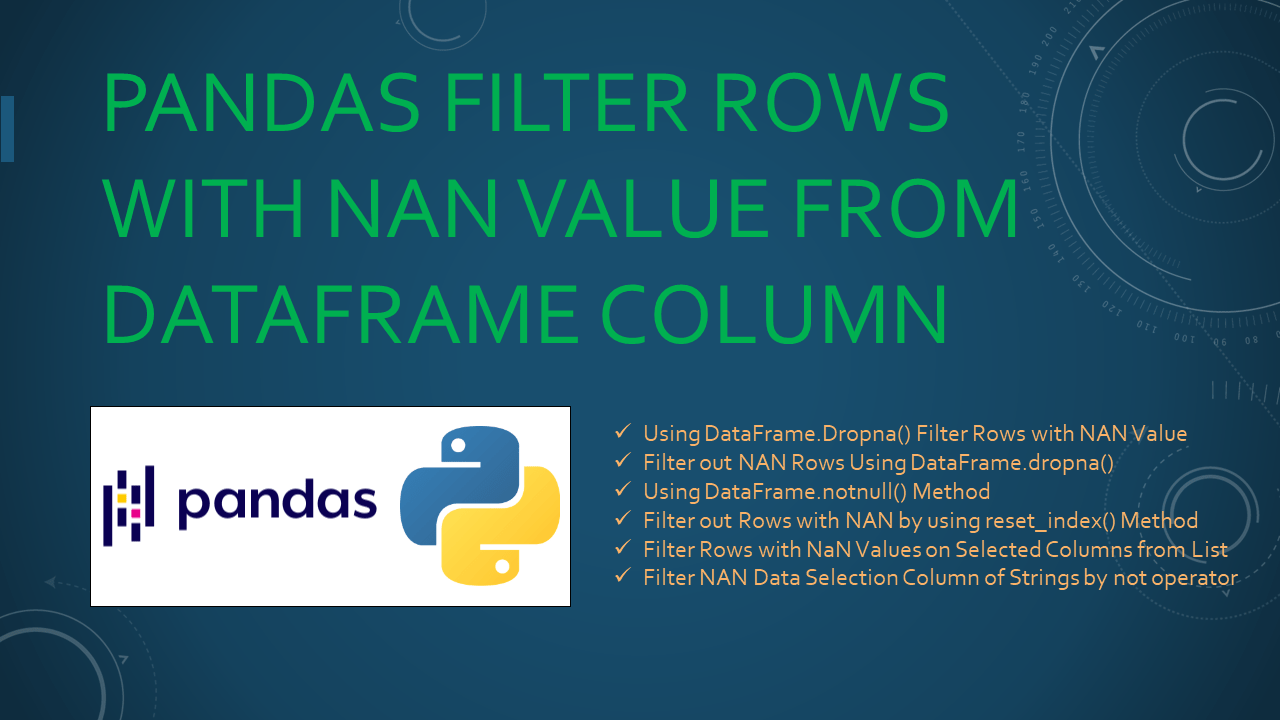# Pandas Filter Rows with NAN Value from DataFrame Column

• Post author:
• Post category:Pandas

You can filter out rows with NAN value from pandas DataFrame column string, float, datetime e.t.c by using `DataFrame.dropna()` and `DataFrame.notnull()` methods. Python doesn’t support Null hence any missing data is represented as None or NaN. NaN stands for `Not A Number` and is one of the common ways to represent the missing value in the data.

In this article, I will explain how to filter out rows with NAN values from the Pandas DataFrame column with some examples.

## 1. Quick Examples Filter out Rows NAN from DataSelection of Column

If you are in a hurry, below are some quick examples of how to ignore rows with NAN from Pandas DataFrame.

``````
# Below are some Quick examples.

# Example 1: Using DataFrame.dropna() method drop all rows that have NAN/none.
df2=df.dropna()

# Example 2: Filter out NAN data selection column by DataFrame.dropna().
df2 = df.dropna(thresh=2)

# Example 3: Pandas find columns with nan to update.
df2=df[df.Duration.notnull()]

# Example 4: Drop rows that has all NaN values.
df2=df.dropna(how='all')

# Example 5: Using reset_index() Method.
df2=df.dropna().reset_index(drop=True)

# Example 6: Two columns by using subset parameter.
df2=df.dropna(subset=['Courses','Fee'])

# Example 7: Filter NAN Data selection column of strings by not operator.
df2 = df[~pd.isnull(df['Courses'])]
``````

Now, let’s create a Pandas DataFrame with a few rows and columns and execute some examples to learn how to drop rows with NAN values. Our DataFrame contains column names `Courses``Fee``Duration`, and `Discount`.

``````
# Create a pandas DataFrame.
import pandas as pd
import numpy as np
technologies= {
'Courses':["Spark","PySpark","Spark","Python","PySpark","Java"],
'Fee' :[22000,25000,np.nan,np.nan,np.nan,np.nan],
'Duration':['30days',np.nan,'30days','N/A', np.nan,np.nan]
}
df = pd.DataFrame(technologies)
print("Create DataFrame:\n", df)
``````

Yields below output.

## 2. Using DataFrame.Dropna() Filter Rows with NAN Value

By using `pandas.DataFrame.dropna()` method you can filter rows with Nan (Not a Number) and None values from DataFrame. Note that by default it returns the copy of the DataFrame after removing rows. If you want to remove it from the existing DataFrame, you should use `inplace=True`

``````
# Using DataFrame.dropna() method drop all rows that have NAN/none.
df2=df.dropna()
print("After filtering the rows with NAN values:\n", df2)
``````

Yields below output.

Alternatively, you can also use `axis=0` as a param to remove rows with NaN, for example `df.dropna(axis=0)`

## 3. Filter out NAN Rows Using DataFrame.dropna()

Filter out NAN rows (Data selection) by using `DataFrame.dropna()` method. The `dropna()` function is also possible to drop rows with NaN values `df.dropna(thresh=2)`it will drop all rows where there are at least two non-NaN.

``````
# Filter out NAN data selection column by DataFrame.dropna().
df2 = df.dropna(thresh=2)
print(df2)
``````

Yields below output.

``````
# Output:
Courses      Fee Duration
0    Spark  22000.0   30days
1  PySpark  25000.0      NaN
2    Spark      NaN   30days
3   Python      NaN      N/A
``````

## 3. Using DataFrame.notnull() Method

The `DataFrame.notnull()` method is used to detect non-missing values for an array-like object. This method takes a scalar or array-like object and indicates whether values are valid.

``````
# Pandas find columns with nan to update.
df2=df[df.Duration.notnull()]
print(df2)
``````

Yields below output.

``````
# Output:
Courses      Fee Duration
0   Spark  22000.0   30days
2   Spark      NaN   30days
3  Python      NaN      N/A
``````

## 4. Filter out Rows with NAN by using reset_index() Method

`reset_index()` method is used to generate a new DataFrame or Series with the index reset. This is useful when the index needs to be treated as a column or it can be reset to the default before another operation.

``````
# Using reset_index() Method.
df2=df.dropna().reset_index(drop=True)
print(df2)

# OutPut:
#   Courses      Fee Duration
# 0   Spark  22000.0   30days
``````

## 5. Filter Rows with NaN Values on Selected Columns from List

In this section, let’s see how to drop rows only when selected columns have NaN/None values in DataFrame, you can achieve this by using `subset` parameter. The subset parameter is simply selecting particular rows and columns of data from a DataFrame (or Series).

``````
# Two columns by using subset parameter.
df2=df.dropna(subset=['Courses','Fee'])
print(df2)
``````

Yields below output.

``````
# Output:
Courses      Fee Duration
0    Spark  22000.0   30days
1  PySpark  25000.0      NaN
``````

Alternatively, Use `how='all'` to remove rows that have all NaN/None values in a row(data is missing for all elements in a row)

``````
# Drop rows that has all NaN values.
df2=df.dropna(how='all')
print(df2)
``````

Yields below output.

``````
# Output:
Courses      Fee Duration
0    Spark  22000.0   30days
1  PySpark  25000.0      NaN
2    Spark      NaN   30days
3   Python      NaN      N/A
4  PySpark      NaN      NaN
5     Java      NaN      NaN
``````

## 6. Filter NAN Data Selection Column of Strings by not operator

Filter NAN Data Selection column of strings by not(~) operator is used to to negate the statement.

``````
# Filter NAN Data selection column of strings by not operator.
df2 = df[~pd.isnull(df['Courses'])]
print(df2)
``````

Yields output same as above.

## 7. Complete Examples to Filter Out Rows with NAN Value

Below is a complete example to filter out rows with NAN values from the DataFrame.

``````
# Complete examples to filter out rows with NAN value.
# Create a pandas DataFrame.
import pandas as pd
import numpy as np
technologies= {
'Courses':["Spark","PySpark","Spark","Python","PySpark","Java"],
'Fee' :[22000,25000,np.nan,np.nan,np.nan,np.nan],
'Duration':['30days',np.nan,'30days','N/A', np.nan,np.nan]
}
df = pd.DataFrame(technologies)
print(df)

# Using DataFrame.dropna() method drop all rows that have NAN/none.
df2=df.dropna()
print(df2)

# Filter out NAN data selection column by DataFrame.dropna().
df2 = df.dropna(thresh=2)
print(df2)

# Pandas find columns with nan to update.
df2=df[df.Duration.notnull()]
print(df2)

# Drop rows that has all NaN values.
df2=df.dropna(how='all')
print(df2)

# Using reset_index() Method.
df2=df.dropna().reset_index(drop=True)
print(df2)

# Two columns by using subset parameter.
df2=df.dropna(subset=['Courses','Fee'])
print(df2)

# Filter NAN Data selection column of strings by not operator.
df2 = df[~pd.isnull(df['Courses'])]
print(df2)
``````

## 8. Conclusion

In this article, You have learned how to filter nan rows from pandas DataFrame by using `DataFrame.dropna()`, `DataFrame.notnull()` methods. Also learned how to filter rows only when all values are NaN/None, only when selected columns have NaN values, and using inplace parameters.

## References